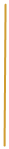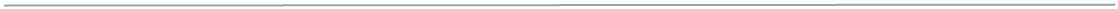Elementary School
Math curriculum

1.Counting strategies and recognizing number
patterns,
2.Determined numbers relation to tens and ones,
3.Solving one -digit addition and subtraction,
4.Understand units of measure.
1.Learn place value to 100,
estimate the solution,
3.Odd and even numbers,
4.Solving multistep word problems,
5.Time, money and primary unit of measure.
1.Multiplication and division,
2.Fraction,
3.Calculating elapsed time,
4.Understand charts and graphs,
5.Multiplying multi-digits numbers and
decimals.
2.Area and perimeter,
3.Comparing, rounding and ordering
decimals and fractions.
1.Calculate quotients, division of multi-
digits numbers with reminders,
2.Mathematical operations on fractions
and decimals,
3.Order of operations,
4.Positive and negative numbers,
5.Surface area.
1.Prime factorization,
2.Ratio and rates,
3.Solving linear equations and inequalities,
4.Math properties, concept of
ϖ(pi),
5.Mean, median and mode.